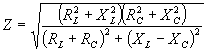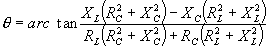## Most Used Formulas (cont.)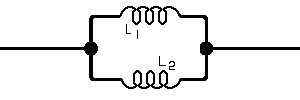or where only 2 inductances L1 and L2 are involved,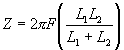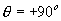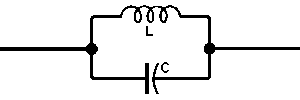of inductance and capacitance in parallel,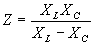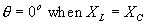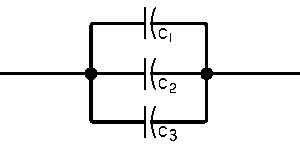of capacitance in parallel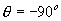or where only 2 capacitances C1 and C2 are involved,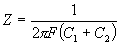of inductance, resistance and capacitance in parallel,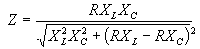of resistance and inductance in parallel,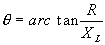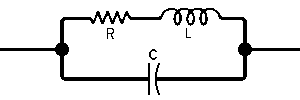of inductance and series resistance in parallel with   capacitance,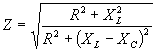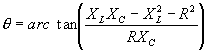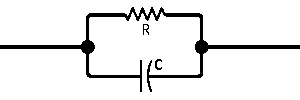of capacitance and resistance in parallel,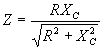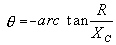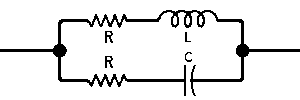of capacitance and series resistance in parallel with   inductance and series resistance,